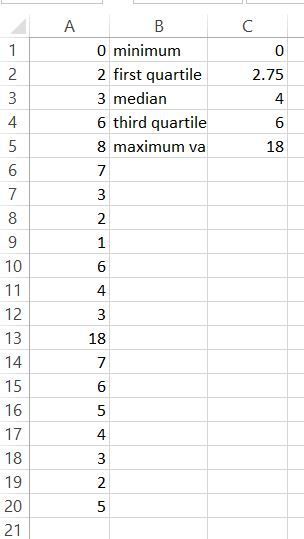### How To Calculate Quartiles in Excel

In this Excel tutorial you will teach yourself how to calculate quartiles.

To calculate quartiles in Excel just use QUARTILE.INC function.

QUARTILE.INC syntax is:

=QUARTILE.INC(array, quart)

where:

quart is a number of quartile which you want to calculate

Example:

Your data table is in A1:A10 range and you want to calculate second quartile.

To calculate second quartile just use this function:

=IFERROR(QUARTILE.INC(A1:A20,2),”No Values”)

IFERROR function in this formula prevents you from #NUM! error. It happens when your data table will be empty.

You can set quart in QUARTILE.INC formula to 0 – 4.

=IFERROR(QUARTILE.INC(A1:A20;0);”No Values”) – shows minimum value

=IFERROR(QUARTILE.INC(A1:A20,1),”No Values”) – calculates first quartile

=IFERROR(QUARTILE.INC(A1:A20,2),”No Values”) – calculates second quartile (which is median)

=IFERROR(QUARTILE.INC(A1:A20,3),”No Values”) – calculates third quartile

=IFERROR(QUARTILE.INC(A1:A20,4),”No Values”) – shows maximum valueTip: QUARTILE.INC function is available since Excel 2010 version. In previous versions of Excel use QUARTILE function. Syntax is the same.

## Template

```Further reading: